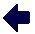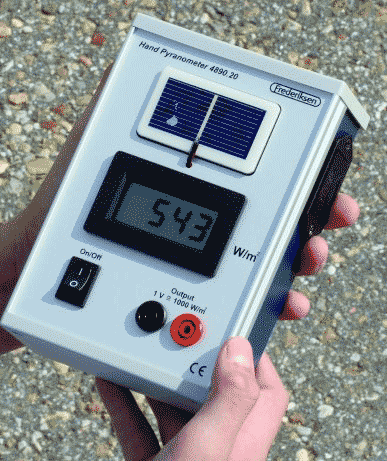# Handheld PyranometerPyranometer, L2-4890.25, £115.50The instrument is designed for field measurements of global solar irradiance.The solar radiation spectrum extends approximately from 300 to 2,800 nm. The unit is supplied with a digital display for direct readout of the irradiance in watts per square meter. Two 4 mm sockets are provided so that the signal can, if required, be used by a datalogger. The device is powered by a 9V battery.

The instrument is very easy to use. It is switched on using the on/off button, the silicon cell detector is oriented to the direction of interest, and the global irradiance in watts per square meter can be read directly from the digital display.
Pyranometers are frequently used in meteorology, climatology, solar energy studies and building physics. They can be seen in many meteorological stations - typically installed horizontally and next to solar panels - typically mounted with the sensor surface in the plane of the panel.

Calibration
Each instrument is individually calibrated in sunlight using a Kipp-Zonen CM21 pyranometer as a reference instrument. Under typical conditions the accuracy of the instrument is within +/-5% of full scale. The precision of the instrument is +/- 1 watt per square meter. The user can make fine adjustments of the calibration if required by means of a trim potentiometer accessible through a small circular aperture on the rear of the instrument.

Technical data
Dimensions: 185 x 108 x 56 mm.
Power supply: 9V battery.
Measuring range: 0-1999 W/m2.
Resolution: 1 W/m2.
Accuracy: +/-5% of full scale.

Sample Application:
The instrument can be used in laboratory exercises and projects to measure the solar global irradiance
in watts per square meter. When measuring the performance of for example a solar cell module, the efficiency of the module can be found. Here is an example:
A solar cell module has an area of 0.5 square meters, and it supplies a voltage of 20 volts and a current of 3.5 amperes when operating at the maximum power point. The hand pyranometer shows that the irradiance is 1000 watts per square meter. The output of the module is then 20 volts times 3.5 amperes = 70 watts, i.e. 140 watts per square meter. The module efficiency is therefore equal to 140 watts divided by 1000 watts per square meter = 14%.>